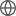Old Web
English
We investigate the slow-motion and weak-field approximation of the general ghost-free parity-violating (PV) theory of gravity in the parametrized post-Newtonian (PPN) framework, and derive the perturbative field equations, which are modified by the PV terms of this theory. The complete PPN parameters are obtained by solving the perturbative field equations. We find that all the PPN parameters are exactly same with those in general relativity, except for an extra parameter $\kappa$, which is caused by the a new crul-type term in the gravitomagnetic sector of the metric in this theory. We calculate the precession effects of gyroscopes in this theory and constrain the model parameters by the observations of Gravity Probe B experiment.